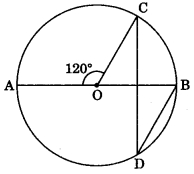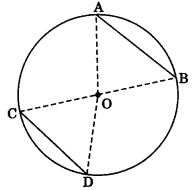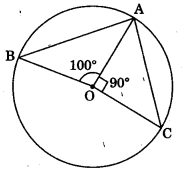# CBSEtips.in

## Saturday, 20 February 2021

### CBSE Class 9 Maths - MCQ and Online Tests - Unit 10 - Circles

#### CBSE Class 9 Maths – MCQ and Online Tests – Unit 10 – Circles

Every year CBSE  schools conducts Annual Assessment exams for 6,7,8,9,11th standards. These exams are very competitive to all the students. So our website provides online tests for all the 6,7,8,9,11th standard’s subjects. These tests are also very effective and useful for those who preparing for any competitive exams like Olympiad etc. It can boost their preparation level and confidence level by attempting these chapter wise online tests.

These online tests are based on latest CBSE syllabus. While attempting these our students can identify the weak lessons and continuously practice those lessons for attaining high marks. It also helps to revise the NCERT textbooks thoroughly.

#### CBSE Class 9 Maths – MCQ and Online Tests – Unit 10 – Circles

Question 1.
In the given figure, AB is a chord of a circle with centre O and AB is produced to C such that BC = OB. Also, CO is joined and produced to meet the circle in D. If ?ACD = 25°, then ?AOD =(a) 50°
(b) 75°
(c) 90°
(d) 100°

Question 2.
In the given figure, O is the centre of a circle and ?AOC = 120°. Then, ?BDC =(a) 60°
(b) 45°
(c) 30°
(d) 15°

Question 3.
AB and CD are two equal chords of a circle with centre O such that ?AOB = 80°, then ?COD =(a) 100°
(b) 80°
(c) 120°
(d) 40°

Question 4.
In the given figure, BOC is a diameter of a circle with centre O. If AB and CD are two chords such that AB || CD and AB = 10 cm, then CD =(a) 5 cm
(b) 12.5 cm
(c) 15 cm
(d) 10 cm

Question 5.
The radius of a circle is 13 cm and the length of one of its chords is 10 cm. The distance of the chord from the centre is
(a) 11.5 cm
(b) 12 cm
(c) $$\sqrt{69}$$ cm
(d) 23 cm

Question 6.
A chord is at a distance of 8 cm from the centre of a circle of radius 17 cm. The length of the chord is
(a) 25 cm
(b) 12.5 cm
(c) 30 cm
(d) 9 cm

Question 7.
In the given figure, AB is a chord of a circle with centre O and BOC is a diameter. If OD ? AB such that OD = 6 cm, then AC =(a) 9 cm
(b) 12 cm
(c) 15 cm
(d) 7.5 cm

Question 8.
In the given figure, O is the centre of a circle. If ?AOB = =100° and ?AOC = 90°, then ?BAC =(a) 85°
(b) 80°
(c) 90°
(d) 75°

Question 9.
In figure, O is the centre of a circle and ?AOB = 140°, then ?ACB =(a) 70°
(b) 80°
(c) 110°
(d) 40°

Question 10.
In figure, O is the centre of a circle and ?AOB = 130°, then ?ACB =(a) 50°
(b) 65°
(c) 115°
(d) 155°

Question 11.
In the given figure, O is the centre of a circle in which ?OAB = 20° and ?OCB = 50°. Then, ?AOC =(a) 50°
(b) 70°
(c) 20°
(d) 60°

Question 12.
In the given figure, O is the centre of a circle in which ?AOC = 100° side AB of quadrilateral OABC has been produced to D. Then, ?CBD =(?) 50°
(b) 40°
(c) 25°
(d) 80°

Question 13.
In the given figure, BOC is a diameter of a circle and AB = AC. Then, ?ABC =(a) 30°
(b) 45°
(c) 60°
(d) 90°

Question 14.
In the given figure, O is the centre of a circle. If ?OAB = 40° and C is a point on the circle, then ?ACB =(a) 40°
(b) 50°
(c) 80°
(d) 100°

Question 15.
In the given figure, O is the centre of a circle and ?OAB = 50°. Then, ?BOD =(?) 130°
(b) 50°
(c) 100°
(d) 80°

Question 16.
In the given figure, ABCD is the cyclic quadrilateral in which BC = CD and ?CBD = 35°. Then, ?BAD =(?) 65°
(b) 70°
(c) 110°
(d) 90°

Question 17.
In figure, ?ABC is an equilateral triangle and ABDC is a quadrilateral then ?BDC =(?) 90°
(b) 60°
(c) 120°
(d) 150°

Question 18.
In figure, sides AB and AD of a quadrilateral ABCD are produced to E and F respectively. If ?CBE = 100°, then find ?CDF.(a) 100°
(b) 80°
(c) 130°
(d) 90°

Question 19.
An equilateral triangle of side 9 cm is inscribed a circle. The radius of the circle is
(a) 3 cm
(b) 3v2 cm
(c) 3v3 cm
(d) 6 cm

Question 20.
In the given figure, ?ABC and ?DBC are inscribed in a circle such that ?BAC = 60° and ?DBC = 50°. then, ?BCD =(a) 50°
(b) 60°
(c) 70°
(d) 80°